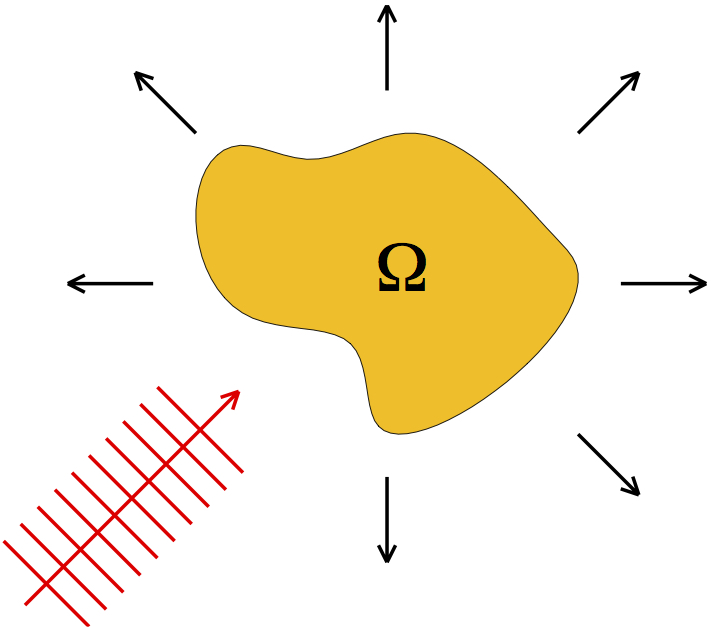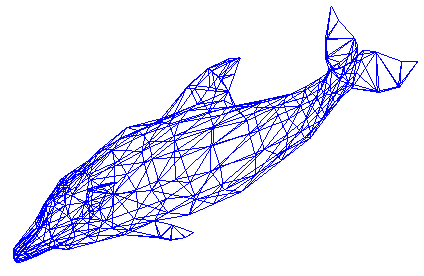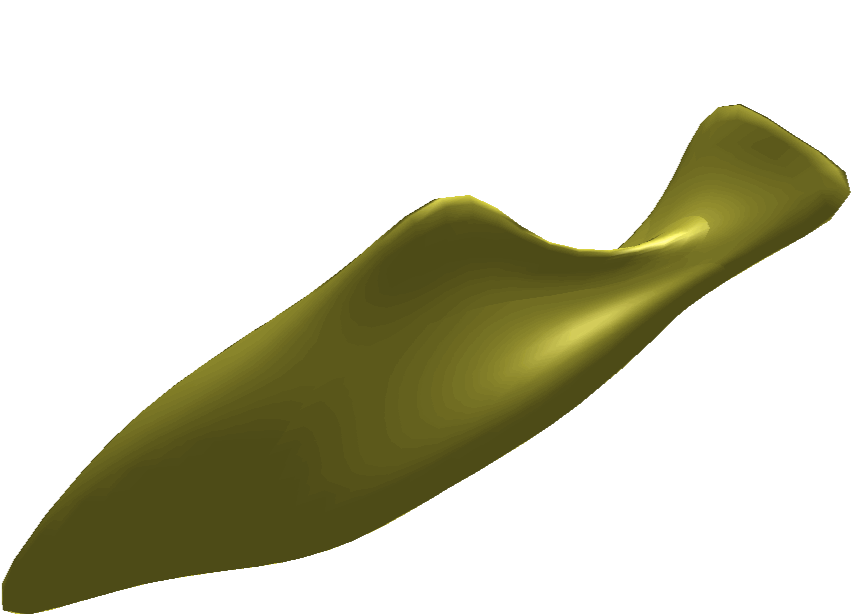# Inverse Obstacle Scattering

It is well known that the propagation of an acoustic wave in a homogeneous, isotropic, and inviscid fluid is approximately described by a velocity potential $$U({\bf x},t)$$ satisfying the wave equation $$U_{tt} = c^2\Delta U$$. Here, $$c$$ denotes the speed of sound, $$v = U_{\bf x}$$ is the velocity field, and $$p = -U_t$$is the pressure. If $$U$$ is time harmonic, that is $$U({\bf x},t) = \operatorname{Re}\big(u({\bf x})e^{-i\omega t}\big)$$, $$\omega>0$$, in complex notation, then the complex-valued space-dependent $$u$$ satisfies the Helmholtz equation $\Delta u + \kappa^2 u = 0\quad\text{in}\ \mathbb{R}^3\setminus\overline{\Omega}.$ The domain $$\Omega\subset\mathbb{R}^3$$ describes an obstacle and $$\kappa = \omega/c$$ is the wave number. We assume that $$\Omega$$ is simply connected and bounded with smooth boundary $$\Gamma = \partial\Omega$$. For sound-soft obstacles, the pressure $$p$$ vanishes on $$\Gamma$$, which leads to the Dirichlet boundary condition $u=0\quad\text{on}\ \Gamma.$We shall consider the situation that $$u = u_i+u_s$$ is composed of a known incident plane wave $$u_i({\bf x}) = e^{i\kappa {\bf d}\cdot{\bf x}}$$ with direction $${\bf d}$$, and a scattered wave $$u_s$$. The scattered field satisfies the Sommerfeld radiation condition $\lim_{r\to\infty} r \left\{ \frac{\partial u_s}{\partial r} - i\kappa u_s\right\} = 0,\quad r = \|{\bf x}\|,$ which implies the asymptotic behaviour $u_s({\bf x}) = \frac{e^{i\kappa\|{\bf x}\|}}{\|{\bf x}\|} \left\{u_\infty\bigg(\frac{\bf x}{\|{\bf x}\|}\bigg) + \mathcal{O}\bigg(\frac{1}{\|{\bf x}\|}\bigg)\right\}, \quad \|{\bf x}\|\to\infty.$ The function $$u_\infty:\mathbb{S}^2 :=\{{\bf x}: \|{\bf x}\|=1\}\to\mathbb{C}$$ is called the far-field pattern.

The inverse obstacle scattering problem reads as follows: Given the direction $${\bf d}$$, the far field pattern $$u_\infty$$ of the scattered wave, find the domain $$\Omega$$. In cooperation with Thorsten Hohage (Georg-August-University of Göttingen, Germany), we are developing efficient methods to solve this severely ill-posed inverse problem. Generating for example synthetic data from the following dolphinwe gain the reconstruction### Selected Publications

1. H. Harbrecht and T. Hohage. Fast methods for three-dimensional inverse obstacle scattering. J. Integral Equations Appl., 19(3):237-260, 2007.

2. H. Harbrecht and T. Hohage. A Newton method for reconstructing non star-shaped domains in electrical impedance tomography. Inverse Probl. Imaging, 3(2):353-371, 2009.